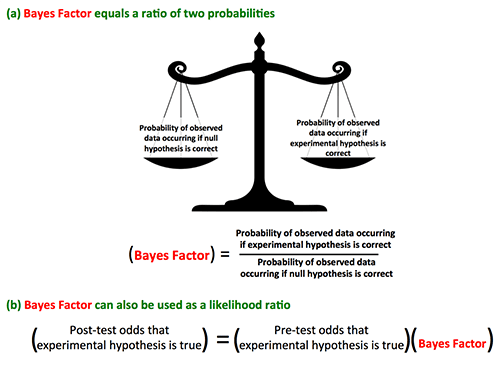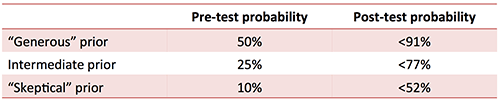A post a few weeks ago calculated the fragility index of the NINDS trial (which turned out to be only three).  Very briefly, the fragility index tests how many events would need to be changed for the p-value to increase above 0.05, rendering the study “statistically insignificant.”  Ryan Radecki commented that he was concerned that the fragility index was married to the p-value, thereby inheriting the flaws of frequentist statistics.  Perhaps we should ditch the p-value and the fragility index, switching instead to a purely Bayesian approach to statistics?

This post will attempt to answer these questions, by exploring the relationship between the fragility index, p-values, and Bayes Factors for a 2×2 table.

### Defining and calculating the maximal Bayes Factor

#### Background on Bayesian Statistics & Bayes Factors

Frequentist statistics is based upon calculating the probability of the observed data occurring if the null hypothesis were true (the p-value).  The problem with this approach is that it tells us little about the probability of the experimental hypothesis being true (if this doesn't make sense, start with this post).

Bayesian statistics takes a broader approach.  In Bayesian statistics, the probability of the observed data occurring is determined both under the null hypothesis and also under the experimental hypothesis (figure below).  The Bayes Factor is the ratio of these probabilities.  This Bayes Factor also functions as a likelihood ratio to calculate the post-experiment odds that the experimental hypothesis is true.Bayesian statistics is a more robust approach, but this comes at a price.  Bayesian statistics haven't yet been broadly applied due to several drawbacks:

• Bayesian statistics classically requires pre-specification of an experimental hypothesis, which may be subjective.
• Bayesian statistics requires a pre-test probability of the experimental hypothesis, which also may add some subjectivity.
• Bayesian statistics often requires very sophisticated mathematical calculations (compared to frequentist statistics, which can often be performed with simple statistical calculators).

#### Maximal Bayes Factor for a 2×2 table

One way to render Bayesian Statistics a bit more objective and within the reach of clinicians is to calculate the maximal Bayes Factor that could be obtained for any experimental hypothesis (1).  By definition, this is a generously high Bayes Factor which will favor the experimental hypothesis.  As such, the maximal Bayes Factor can be useful for defining the limits of what the study cannot prove (2).  For a 2×2 table, the maximal Bayes Factor is calculated with this equation (where x is equal to the value of the chi-test statistic)(3).### Relationship between maximal Bayes Factor and the p-value.

For a 2×2 table, the p-value can be calculated using a chi-square distribution with one degree of freedom (4).  Since both the p-value and the maximal Bayes Factor are calculated from the chi-squared statistic, they can be directly plotted against each other:A few observations are in order:

• A lower p-value correlates with a higher maximal Bayes Factor.
• A p-value of 0.05 correlates with a maximal Bayes Factor of 8.   This isn't decisive.   For example, a Bayes Factor of 8 would only boost an experimental hypothesis from a pre-test probability of 50% to a post-test probability of 89%.  Of course, in all likelihood the Bayes Factor corresponding to p=0.05 would substantially below 8, yielding a post-test probability well under 89%.  This illustrates how using a cutoff of p<0.05 leads to false-positive research findings which cannot be replicated.

### Integrating maximal Bayes Factor, p-value, and the Fragility Index

The close relationship between the maximal Bayes Factor and the p-value implies that Fragility must be related to both.  The key here is really how robust the chi-squared test statistic is to changes in the data (because both the p-value and the maximal Bayes Factor ultimately depend on the chi-squared parameter).  The chi-squared statistic tends to be more labile when fewer numbers of events are observed, leading to fragility in both the p-value and the maximal Bayes factor.

#### Reformulating Fragility for Bayesian analysis

Consider two studies which both yield a Bayes Factor of 6.  In the first study, if the outcome of one patient is changed, the Bayes Factor decreases from 6 to 4.  In the second study, if the outcome of one patient is changed, the Bayes Factor decreases from 6 to 5.8.  Clearly the first study is more “fragile” than the first study.

Bayesian statistics doesn't yield a single binary outcome (i.e. p<0.05) the way that Frequentist statistics does.  Thus, it may be less clear how exactly to quantify Fragility in a Bayesian statistical system.  One approach could be the deviation in the Bayes Factor if a single patient outcome is changed.

Regardless of exactly how it is expressed, some description of Fragility should be useful regardless of which statistical approach is being utilized (Bayesian or Frequentist).   Furthermore, the fragility of a dataset should be similar regardless of which statistical approach is being utilized.   For example, if a study is fragile using a Frequentist analysis, it should also be fragile using a Bayesian analysis.

### Example: The NLST trial of CT screening for detection of lung cancer

Current guidelines recommend lung cancer screening with CT scans, based almost exclusively on a single trial:  the NLST trial from 2011.  This was a prospective multi-center RCT which randomized 53,454 patients to receive lung cancer screening with chest X-rays vs. CT scans.

It's controversial whether the endpoint of screening trials should be disease-specific mortality or all-cause mortality (Penston 2011).  Screening often manages to reduce disease-specific mortality, without meeting the more robust outcome of reducing all-cause mortality.  The NLST trial chose disease-specific mortality as its primary endpoint.  The results were interpreted as showing a reduction in both disease-specific and all-cause mortality, providing strong support for the implementing CT screening:Let's evaluate the data from this study regarding whether lung cancer screening reduces all-cause mortality. The following 2×2 table relates lung cancer screening with mortality:##### (1) Traditional analysis

The chi-square statistic for this study is 4.16, yielding a p-value of 0.041 (5).  This p-value is below the standard cutoff of 0.05, so the traditional interpretation of this data is that it shows a statistically significant reduction in all-cause mortality.

##### (2) Fragility Index analysis

Fragility Index for all-cause mortality is five (e.g. using the Fragility Index Calculator).

The instability index can be calculated as follows:

Instability Index = (Effects from baseline imbalance) + (Post-randomization crossover) + (Loss to followup)

• There is no discernible baseline imbalance.
• Post-randomization crossover: ~4% of patients in the control group crossed over (equal to ~1100 patients)
• Loss to followup: ~3% of patients were lost to follow-up (equal to ~1600 patients).

Overall the instability index might be estimated to be ~2700 patients.  Given that the instability index is far greater than the fragility index, the finding that CT scans improve all-cause mortality appears quite fragile.

##### (3) Maximal Bayes Factor analysis

These results have a maximal Bayes Factor of 9.9.  Here is where things get tricky. In order to determine the probability that CT scans reduce all-cause mortality, we must first determine the pre-test probability that this is true.  This is often impossible, so typically a few pre-test probabilities are used.  In this case, the following may be used:

• Pre-test probability of 50%:  This may be selected in an attempt to establish fairness (a “blind” prior probability).  Given the difficulties which must be overcome to successfully screen for lung cancer, I think 50% is a generous pre-test probability.  Furthermore, most cancer screening programs have failed to reduce all-cause mortality, so a 50% pre-test probability is arguably quite high.
• Pre-test probability of 25%:  This might be a reasonable choice.
• Pre-test probability of 10%:  This might be a “skeptical” prior probability, perhaps representing the perspective of someone who had encountered many patients with iatrogenic complications from lung nodule management.

Based on a Bayes Factor <9.9 and these pre-test probabilities, we can now calculate the post-test probabilities:Even with the most generous prior probability, the post-test probability must be below 91%.  Thus, this study cannot establish that an all-cause mortality benefit exists with >95% certainty.  More realistically, the post-test probability is probably about 60-80%, so this data isn't close to being definitive.  Thus the widespread claim that lung cancer screening “saves lives” may not be true (6).

### Where to go from here:  Balancing skepticism, optimism, and nihilism

The fragility index and Bayesian analysis suggest that medical evidence isn't nearly as robust as we thought.  For example, clinical studies which are considered the bedrock of modern medicine (e.g. the NINDS trial) may actually be fragile and non-definitive.  Smaller trials are often worse.  It quickly becomes clear why medicine continues to suffer from reversals in practice.

This may be disconcerting.  However, from the standpoint of any individual practitioner, it may not make a huge difference.  We must address clinical scenarios with the best evidence available.  Whether that evidence is perfect or imperfect, we don't have the luxury to wait for better evidence.  In this context, it is often sensible to base therapy on treatments which are fragile or have borderline statistical significance.

The situation is reversed when considering evidence-based guidelines.  Premature acceptance of an ineffective therapy into guidelines may have profoundly negative consequences.  First, this discourages further research into the therapy, leading to inertia.  Second, physicians and hospitals may feel obligated to adhere to this therapy which is suddenly considered the “standard of care.”  Finally, future therapies may be tested in addition to, rather than in comparison to the ineffective therapy.  Eventually this creates a complex treatment regimen resembling a house of cards.• A 2×2 table with a p-value of 0.049 represents only moderate evidence supporting the experimental hypothesis (equivalent to a likelihood ratio below 8).  Thus, the concept that p<0.05 equals a “statistically significant” result is mathematically wrong.
• Maximal Bayes Factor is an easily calculated parameter which can be used to define the limits of what a data set cannot prove.
• The concept of the fragility index can be extended from Frequentist statistics to Bayesian statistics.
• Applying Bayesian statistics may cause reversal of conclusions obtained using Frequentist statistics.
• It is time to debunk the domination of the p-value:  whenever possible, p-values should be accompanied by other metrics, including a fragility index and Bayesian parameters.  No single statistic tells the whole story.
##### Notes
1. Most articles calculate the minimum Bayes Factor, which is the inverse of the maximal Bayes Factor. These are essentially the same thing – two ways of expressing the same evidence strength. I prefer the maximal Bayes Factor because I think it's easier for me to think about a “likelihood ratio of +15” instead of a “likelihood ratio of 0.067.”
2. Make no mistake, this isn't intended as a replacement for full-fledged Bayesian analysis by a statistician trained in Bayesian methods. However, in the absence of such analysis, the maximal Bayes Factor may serve as a bit of a statistical crutch. A more complete Bayesian analysis would be useful to establish what a study can
3. Note that if Chi-squared is less than one, then the Maximal BF is set equal to one. In this scenario, the evidence is quite consistent with the null hypothesis. The references for this formula are: Johnson VE, J.R. Stat Soc B 2005; 67:689 and Held 2010.
4. This is assuming that the sample sizes are large enough that a Yates correction or Fisher Exact test isn't needed.
5. The p-value reported in the manuscript (p=0.02) differs slightly from the p-value obtained from a chi-square test. This isn't an uncommon phenomenon, wherein different statistical tests yield slightly different results. Incidentally, this illustrates why it is illogical to use any single cutoff (i.e. p=0.05) to make a binary decision of statistically significant or insignificant.
6. Whether or not it is beneficial to perform CT screening for lung cancer is beyond the scope of this post. However, it cannot be concluded that CT screening reduces all-cause mortality based on this data.
Social Me